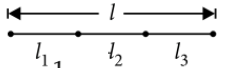Standing waves
Question

# If  are the fundamental frequencies of three segments into which a string is divided, then the original fundamental frequency n of the string is given by

Moderate
Solution

##${n}_{1}=\frac{1}{2{l}_{1}}\sqrt{\frac{T}{\mu }}$                       . . . .  (i)${n}_{3}=\frac{1}{2{l}_{3}}\sqrt{\frac{T}{\mu }}$                          . . . . . (iii) and$n=\frac{1}{2l}\sqrt{\frac{T}{\mu }}$                        . . . . . (iv)$\frac{1}{n}=\frac{2l}{\sqrt{\frac{T}{\mu }}}$$=\frac{1}{{n}_{1}}+\frac{1}{{n}_{2}}+\frac{1}{{n}_{3}}$ (using (i), (ii) and (iii))

Get Instant Solutions